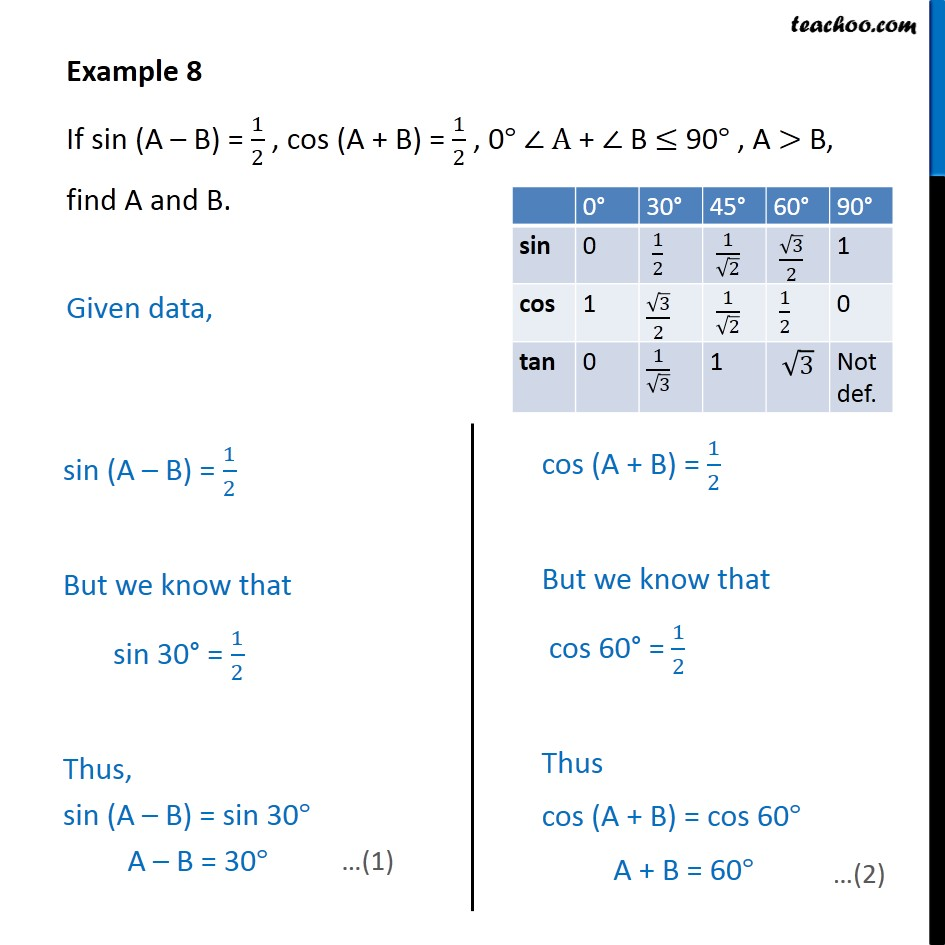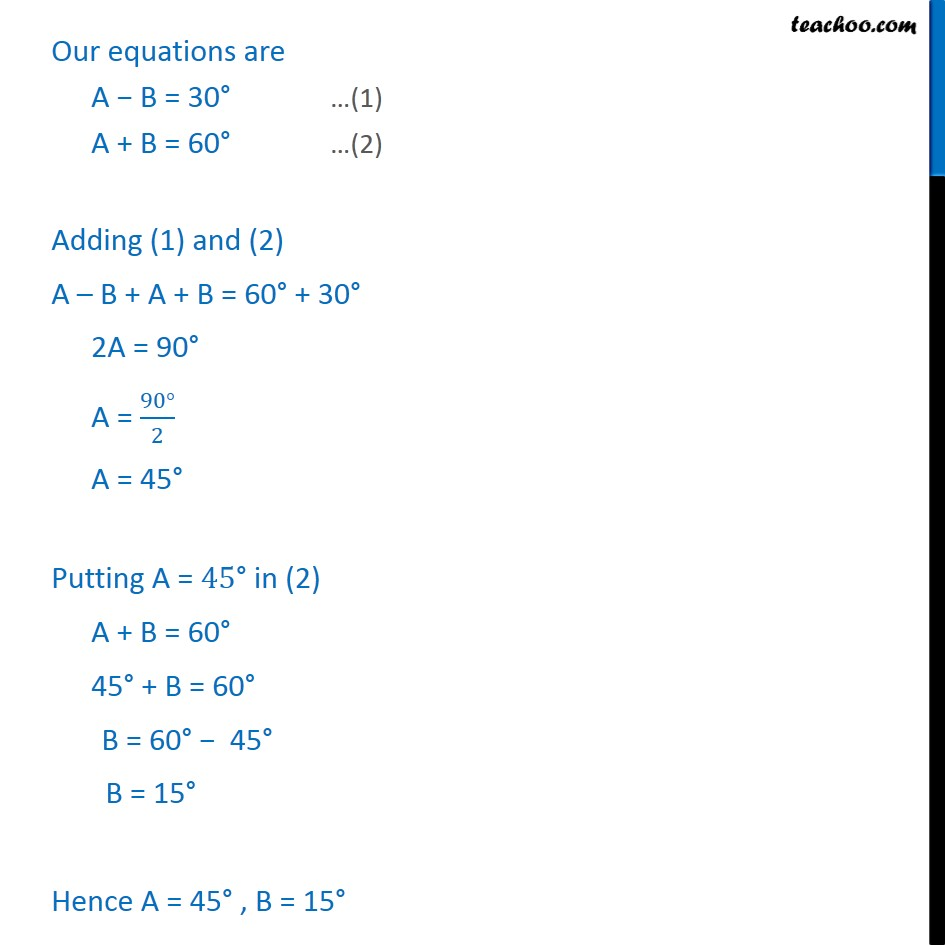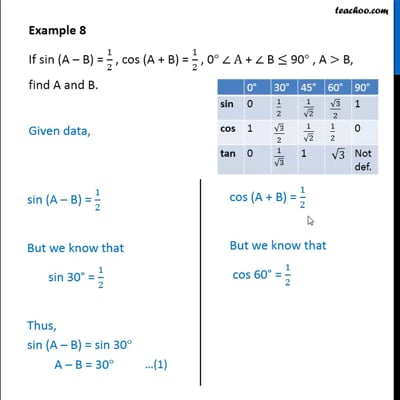Examples

Chapter 8 Class 10 Introduction to Trignometry (Term 1)
Serial order wiseThis video is only available for Teachoo black users

### Transcript

Example 8 If sin (A – B) = 1/2 , cos (A + B) = 1/2 , 0° ∠ A + ∠ B ≤ 90° , A > B, find A and B. Given data, Our equations are A − B = 30° …(1) A + B = 60° …(2) Adding (1) and (2) A – B + A + B = 60° + 30° 2A = 90° A = (90°)/2 A = 45° Putting A = "45°" in (2) A + B = 60° 45° + B = 60° B = 60° − 45° B = 15° Hence A = 45° , B = 15°